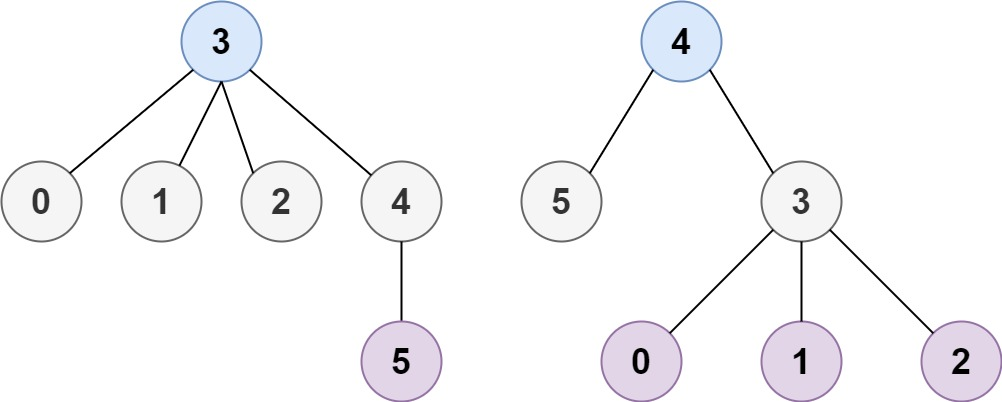310. Minimum Height Trees

A tree is an undirected graph in which any two vertices are connected by exactly one path. In other words, any connected graph without simple cycles is a tree.

Given a tree of `n` nodes labelled from `0` to `n - 1`, and an array of `n - 1` `edges` where `edges[i] = [ai, bi]` indicates that there is an undirected edge between the two nodes `ai` and `bi` in the tree, you can choose any node of the tree as the root. When you select a node `x` as the root, the result tree has height `h`. Among all possible rooted trees, those with minimum height (i.e. `min(h)`)  are called minimum height trees (MHTs).

Return a list of all MHTs' root labels. You can return the answer in any order.

The height of a rooted tree is the number of edges on the longest downward path between the root and a leaf.

Example 1:```Input: n = 4, edges = [[1,0],[1,2],[1,3]]
Output: 
Explanation: As shown, the height of the tree is 1 when the root is the node with label 1 which is the only MHT.
```

Example 2:```Input: n = 6, edges = [[3,0],[3,1],[3,2],[3,4],[5,4]]
Output: [3,4]
```

Example 3:

```Input: n = 1, edges = []
Output: 
```

Example 4:

```Input: n = 2, edges = [[0,1]]
Output: [0,1]
```

Constraints:

• `1 <= n <= 2 * 104`
• `edges.length == n - 1`
• `0 <= ai, bi < n`
• `ai != bi`
• All the pairs `(ai, bi)` are distinct.
• The given input is guaranteed to be a tree and there will be no repeated edges.

310. Minimum Height Trees
``````struct Solution;
use std::collections::VecDeque;
impl Solution {
fn find_min_height_trees(n: i32, edges: Vec<Vec<i32>>) -> Vec<i32> {
let n = n as usize;
if n == 1 {
return vec!;
}
let mut graph: Vec<Vec<usize>> = vec![vec![]; n];
let mut visited: Vec<bool> = vec![false; n];
let mut degree: Vec<usize> = vec![0; n];
for e in edges {
let u = e as usize;
let v = e as usize;
graph[u].push(v);
graph[v].push(u);
degree[u] += 1;
degree[v] += 1;
}

let mut leaves: VecDeque<usize> = VecDeque::new();
for i in 0..n {
if graph[i].len() == 1 {
leaves.push_back(i);
}
}
let mut m = n;
while m > 2 {
m -= leaves.len();
for _ in 0..leaves.len() {
let u = leaves.pop_front().unwrap();
visited[u] = true;
for &v in &graph[u] {
if !visited[v] {
degree[v] -= 1;
if degree[v] == 1 {
leaves.push_back(v);
}
}
}
}
}
leaves.into_iter().map(|x| x as i32).collect()
}
}

#[test]
fn test() {
let n = 4;
let edges = vec_vec_i32![[1, 0], [1, 2], [1, 3]];
let mut res = vec!;
let mut ans = Solution::find_min_height_trees(n, edges);
ans.sort_unstable();
res.sort_unstable();
assert_eq!(ans, res);
let n = 6;
let edges = vec_vec_i32![[0, 3], [1, 3], [2, 3], [4, 3], [5, 4]];
let mut res = vec![3, 4];
let mut ans = Solution::find_min_height_trees(n, edges);
ans.sort_unstable();
res.sort_unstable();
assert_eq!(ans, res);
let n = 3;
let edges = vec_vec_i32![[0, 1], [0, 2]];
let mut res = vec!;
let mut ans = Solution::find_min_height_trees(n, edges);
ans.sort_unstable();
res.sort_unstable();
assert_eq!(ans, res);
}
``````